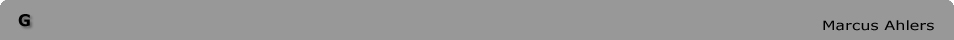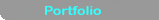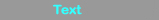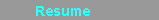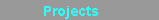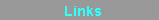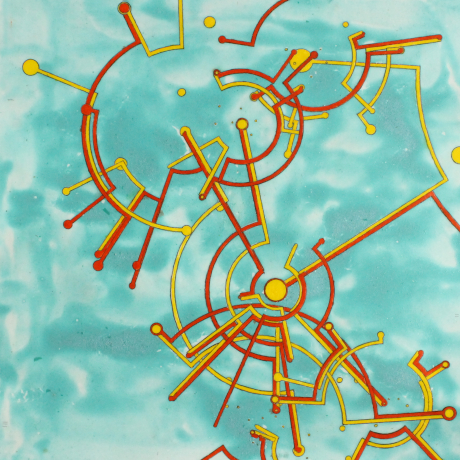Paper, pencil, watercolor, copper carbonate, rubber 37cm x 37cm 2020 click für Deutschen Text The drawing G is part of a series of works using a copper carbonate solution. This material is obtained through electrolysis in water with copper electrodes. In this piece, the orange and yellow lines were derived algorithmically. The process was designed to explore emergent patterns in dynamic systems. Parameters defined as boundary conditions allowed for a significant degree of chance. The algorithm was used to generate nodes with varying randomly determined potentials for connecting with other nodes. The patterns emerged as documentation of the connections between nodes. The algorithm based approach was designed to be sufficiently abstract to relate in base form to diverse systems, yet with a structure selected to attempt articulation of patterns and structures inherent to systems constructing the human experience. 7 trials were performed. Within each trial, 16 nodes were defined according to randomly selected numerical value between 0 and 1 and an angle between 0° and 360°. The numerical values were scaled according to a randomly selected scaling factor between 0,5 and 3,5. The nodes of each trial were then drawn as dots around a central point unique to that trial. The positions were dependent on the angle and the radius. The radius was determined by the numerical values. These numerical values determined for each node can be understood as innate probabilities for connecting with other nodes. Nodes with a higher value were more likely to connect than those with a lower value. 2 rounds of connectivity occurred within each trial. For each round, the innate value of each node was multiplied by another randomly determined numerical value between 0 and 1. This value can be understood as the situational probability of connecting based on the conditions of each round. The diameter of the dot was proportional to the product of the innate and situational probability values. The nodes were listed in descending order according to the products of these two values for each round. Pairs of neighboring nodes in each of these resulting lists were connected, using a yellow line for the first round and an orange line for the second round. Certain nodes did not have the opportunity to connect in a given round. This was determined by a factor NCN (Number of Connecting Nodes) with a value between 8 and 16 and a second factor CF (Cutoff Factor) between 0 and 1 which defined respectively the number of nodes connecting in the given round and a value below which no nodes would connect. Either of these limiting agents were sufficient to independently limit connectivity in the given round. The paths of connectivity were drawn radially from each contributing node inwards to the smaller of the two products defining the connectivity of the nodes for that particular round. Then the shorter path around the circumference was selected to connect the nodes. The nodes with the largest largest initial numerical values, with the highest innate probability to connect and at the furthest distance from the center of a given round, were used as new central points for subsequent trials.